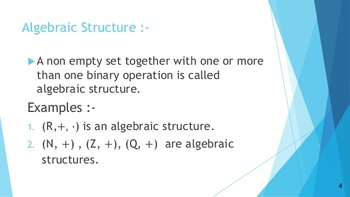# Algebraic structure in elementary group theory﻿Algebraic Structure:﻿

A set with one or more binary operations gives rise to what is commonly known as a algebraic structure. In particular ,the set of integers under the addition operation '+' is an algebraic structure .It is commonly denoted by (Z,+). In the same way ,the set of rational numbers Q under the usual multiplication operation '*',and denoted by (Q,*),is another algebraic structure .A more complicated algebraic structure is the set of real numbers R together with the two usual operations: addition  '+' and multiplication '*'  . Such an algebraic  structure is denoted by (R,+,*).Algebraic structures with one or more binary operations are given special names depending upon additional properties involved.

An algebraic structure consisting of a set G under an operation * on G, and  denoted by(G,*), may enjoy one or more of the following characteristics :

given a,b,c,..... as the  elements of the set G, the algebraic structure (G,*) may be

1. Closed if a*b belongs to G for each a,b belonging to G.

2. Commutative if a*b =b*a belongs to G, for each a,b belonging to G.

3.  Associative if (a*b)*c =a*(b*c), for each a,b,c belonging to G.

4. Existence of identity element: For each 'a' belonging to G, if there exists an element 'e' belonging to G, such that a*e=a=e=e*a then 'e' is called the identity element.

5. Existence of the inverse element : For each 'a' belonging to G, if there exists an element a' belonging to G such that a*a'=e=a'*a then a' is called the inverse of the element a.